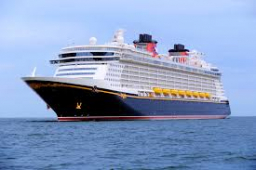Here are

Here are white, blue, and red boats in a marina. Two-thirds of the boats in the marina are white, 4/7 of the remaining boats are blue, and the rest are red. If there are 15 red boats, how many boats are in the marina?

Which tape diagram represents how many of the boats are white?

n =  105

Step-by-step explanation:Did you find an error or inaccuracy? Feel free to write us. Thank you!Tips to related online calculators
Need help to calculate sum, simplify or multiply fractions? Try our fraction calculator.
Do you have a linear equation or system of equations and looking for its solution? Or do you have a quadratic equation?